# Coax Loss due to Dielectric Conduction

Click here to go to our main page on coax loss calculations

Click here to go to our main page on transmission line losses

Click here to go to a more exact metal loss calculation for coax!

Click here to review the derivation for coax loss due to loss tangent

New page for March 2007! Loss due to conduction in coax is the only loss mechanism that acts independent of frequency, and independent of geometry! The second point is not intuitive, but here we'll show you why it's true.

In most cases, the conductivity of a dielectric is so low that this term can almost universally be ignored. PTFE is often used as a dielectric in cables, its resistivity is around 1018 ohm centimeters! (We don't have to tell you that resistivity is the reciprocal of conductivity, do we? Engineers can never seem to make up their minds which one to use...) But with the advent of micromachined structures on semiconductor substrates like bulk silicon, you never know when knowledge of this calculation might come in handy.

Did we mention that extremely expensive EDA software such as Agilent's ADS doesn't feature the contribution of dielectric conductivity in their coax models? We can't explain why that is, but our coax download does it all, check it out!

The first thing to do is to calculate the the conduction per unit length, G'. Here, sigma is the bulk conductivity of the dielectric. The calculation for G' is nearly identical to the calculation for C', the capacitance per unit length, in both cases you are integrating the bulk property from one annular surface to another which results in a ln(D/d) term.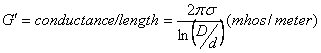The attenuation constant due to conduction is then: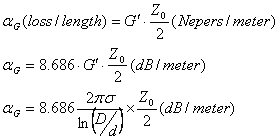A interesting thing happens when you plug in the formula for coax characteristic impedance to the above equation. The most exact equation for coax Z0 is: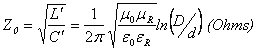Plugging the previous two equations together we get: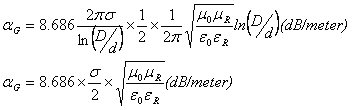Notice that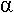G is proportional to ln(D/d), while Z0 is inversely proportional to it. So as G' increases, Z0 decreases (and vice versa).

Canceling the ln(D/d) terms, simplifying the equation, and noting that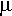R is close enough to one for any material that you'd ever consider for a dielectric so you can ignore it, you are left with: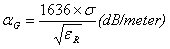ThusG is not a function of coax geometry, it is only a function of two bulk material properties of the dielectric, its conductivity and dielectric constant.

As a final note on this topic, if you calculate the loss of PTFE due to dielectric conduction, you'll soon see that a PTFE coax cable from here to Mars has less than a dB of conduction loss (approximately 10-13 dB/m)! On the other have a coax connection using low resistivity silicon will have loss greater than one dB per millimeter length!